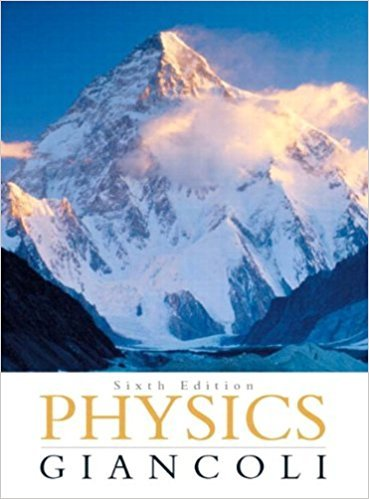×
×

# Answer: Calculate the centripetal acceleration of theISBN: 9780321569837 99

## Solution for problem 3 Chapter 5

Physics: Principles with Applications | 6th Edition

• Textbook Solutions
• 2901 Step-by-step solutions solved by professors and subject experts
• Get 24/7 help from StudySoup virtual teaching assistantsPhysics: Principles with Applications | 6th Edition

4 5 1 311 Reviews
23
2
Problem 3

Calculate the centripetal acceleration of the Earth in its orbit around the Sun. and the net force exerted on the Earth. What exerts this force on the Earth? Assume that the Earth's orbit is a circle of radius 50 x 10M m. [Hint: see the Tables inside the front cover of this book.)

Step-by-Step Solution:
Step 1 of 3
Step 2 of 3

Step 3 of 3

##### ISBN: 9780321569837

This textbook survival guide was created for the textbook: Physics: Principles with Applications, edition: 6. The full step-by-step solution to problem: 3 from chapter: 5 was answered by , our top Physics solution expert on 09/09/17, 04:43AM. Since the solution to 3 from 5 chapter was answered, more than 300 students have viewed the full step-by-step answer. Physics: Principles with Applications was written by and is associated to the ISBN: 9780321569837. This full solution covers the following key subjects: EARTH, orbit, Force, acceleration, calculate. This expansive textbook survival guide covers 33 chapters, and 4141 solutions. The answer to “Calculate the centripetal acceleration of the Earth in its orbit around the Sun. and the net force exerted on the Earth. What exerts this force on the Earth? Assume that the Earth's orbit is a circle of radius 50 x 10M m. [Hint: see the Tables inside the front cover of this book.)” is broken down into a number of easy to follow steps, and 53 words.

Unlock Textbook Solution